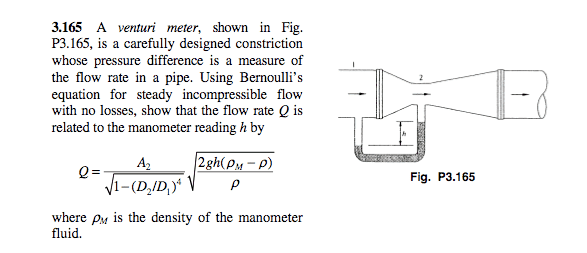# Venturi Meter Finding Pressure Difference

## Homework StatementI guess I am having a hard time seeing what that manometer is doing.

I assume that as fluid moves in at 1 it causes a displacement of the manometer fluid by some distance h.

Now, are we assuming that the pressure is uniform throughout the diameter of the pipe? I think we are, otherwise, I do not see how the manometer reading would be a reflection of the pipe pressure.

So, that being said, the pressure increase on the left side of the manometer is p1 and the pressure decrease on the right side of the manometer is p2.

Good?

So, if the pressure in the pipe=p1=the pressure at some depth h into the manometer and the same for p2, then we have:

$p_1=\rho_mgh$ and $$p_2=\rho_1gh[/itex] thus, $p_1-p_2=gh(\rho_m-\rho_1)$ ## Answers and Replies tiny-tim Science Advisor Homework Helper Hi Saladsamurai!Now use https://www.physicsforums.com/library.php?do=view_item&itemid=115" (which is basically KE + PE = constant), and Q = v1A1 = v2A2Last edited by a moderator: Hey tiny-timI guess I still don't like what I have so far. I know that it is correct, but only because I already knew what formula for a venturi meter I supposed to arrive at. That is, when I originally derived this, I had the densities reversed, i.e., [tex]p_1-p_2=gh(\rho_1-\rho_m)$$

If you call the interface on the left hand side of the manometer (where the pipe fluid meets the manometer fluid) point A,

and the interface on the RHS of the manometer (where the manometer fluid meets the lower pressure fluid) point B:

then p1=pA and p2=pB right?

I am just trying to sort this out in my head.

Because it seems like then pA=h*g*rho_1 and errr...yeah I am lost nowtiny-tim
Homework Helper
(have a rho: ρ)

I don't really understand what you're askingThe pressure difference is gh(ρM - ρ) …

now write out the two equations(have a rho: ρ)

I don't really understand what you're askingThe pressure difference is gh(ρM - ρ) …

now write out the two equationsWhat I am asking, is why the pressure difference is

gh(ρM - ρ)

For some reason I am not seeing it as that. I am seeing it as

gh(ρ - ρM)That's what this is all about

If you call the interface on the left hand side of the manometer (where the pipe fluid meets the manometer fluid) point A,

and the interface on the RHS of the manometer (where the manometer fluid meets the lower pressure fluid) point B:

then p1=pA and p2=pB right?

rl.bhat
Homework Helper

## Homework Statement

So, if the pressure in the pipe=p1=the pressure at some depth h into the manometer and the same for p2, then we have:

$p_1=\rho_mgh$ and [tex]p_2=\rho_1gh[/itex]

thus, $p_1-p_2=gh(\rho_m-\rho_1)$
You have misunderstood about p1 and p2. Let p1 and p2 are the pressure along the common axis of the tubes. At any point on the axis it is same in all directions. When the liquid is not flowing, p1 = p2.. p1 and p2 does not depend on the gravitational force, but they depend on the velocity of the liquid.
Since liquid is moving from left to right, p1 > p2.
The mercury level at A in the left arm is at rest. That means at the same level in both arms, pressure must be the same.
Now proceed to calculate the pressure difference.

•mrreja